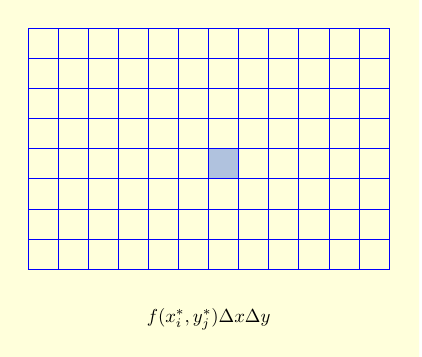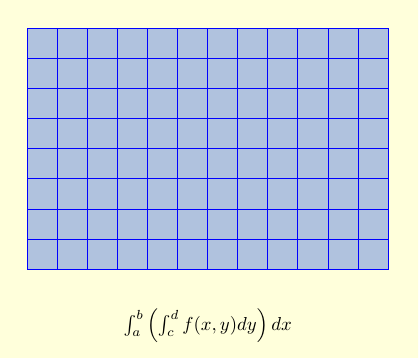Home

#### The Fundamental Theorem of Calculus

Three Different Quantities
The Whole as Sum of Partial Changes
The Indefinite Integral as Antiderivative
The FTC and the Chain Rule

#### The Indefinite Integral and the Net Change

Indefinite Integrals and Anti-derivatives
A Table of Common Anti-derivatives
The Net Change Theorem
The NCT and Public Policy

#### Substitution

Substitution for Indefinite Integrals
Revised Table of Integrals
Substitution for Definite Integrals

#### Area Between Curves

The Slice and Dice Principle
To Compute a Bulk Quantity
The Area Between Two Curves
Horizontal Slicing
Summary

#### Volumes

Slicing and Dicing Solids
Solids of Revolution 1: Disks
Solids of Revolution 2: Washers
Volumes Rotating About the $y$-axis

Behind IBP
Examples
Going in Circles

#### Integrals of Trig Functions

Basic Trig Functions
Product of Sines and Cosines (1)
Product of Sines and Cosines (2)
Product of Secants and Tangents
Other Cases

#### Trig Substitutions

How it works
Examples
Completing the Square

#### Partial Fractions

Introduction
Linear Factors
Improper Rational Functions and Long Division
Summary

#### Strategies of Integration

Substitution
Integration by Parts
Trig Integrals
Trig Substitutions
Partial Fractions

#### Improper Integrals

Type I Integrals
Type II Integrals
Comparison Tests for Convergence

#### Differential Equations

Introduction
Separable Equations
Mixing and Dilution

#### Models of Growth

Exponential Growth and Decay
Logistic Growth

#### Infinite Sequences

Close is Good Enough (revisited)
Examples
Limit Laws for Sequences
Monotonic Convergence

#### Infinite Series

Introduction
Geometric Series
Limit Laws for Series
Telescoping Sums and the FTC

#### Integral Test

The Integral Test
When the Integral Diverges
When the Integral Converges

#### Comparison Tests

The Basic Comparison Test
The Limit Comparison Test

#### Convergence of Series with Negative Terms

Introduction
Alternating Series and the AS Test
Absolute Convergence
Rearrangements

The Ratio Test
The Root Test
Examples

#### Strategies for testing Series

List of Major Convergence Tests
Examples

#### Power Series

Finding the Interval of Convergence
Other Power Series

#### Representing Functions as Power Series

Functions as Power Series
Derivatives and Integrals of Power Series
Applications and Examples

#### Taylor and Maclaurin Series

The Formula for Taylor Series
Taylor Series for Common Functions
Adding, Multiplying, and Dividing Power Series
Miscellaneous Useful Facts

#### Applications of Taylor Polynomials

What are Taylor Polynomials?
How Accurate are Taylor Polynomials?
What can go Wrong?
Other Uses of Taylor Polynomials

#### Partial Derivatives

Definitions and Rules
The Geometry of Partial Derivatives
Higher Order Derivatives
Differentials and Taylor Expansions

#### Multiple Integrals

Background
What is a Double Integral?
Volumes as Double Integrals

#### Iterated Integrals over Rectangles

One Variable at the Time
Fubini's Theorem
Notation and Order

#### Double Integrals over General Regions

Type I and Type II regions
Examples
Order of Integration
Area and Volume Revisited

### One Variable at the Time

Now that we know what double integrals are, we can start to compute them. The key idea is: One variable at a time!

In order to integrate over a rectangle $[a,b] \times [c,d]$, we first integrate over one variable (say, $y$) for each fixed value of $x$. That's an ordinary integral, which we can do using the fundamental theorem of calculus. We then integrate the result over the other variable (in this case $x$), which we can also do using the fundamental theorem of calculus. So a 2-dimensional double integral boils down to two ordinary 1-dimensional integrals, one inside the other. We call this an iterated integral.

There are two ways to see the relation between double integrals and iterated integrals. In the bottom-up approach, we evaluate the sum $$\sum_{i=1}^m \sum_{j=1}^n f\left(x_{i}^*,y_{j}^*\right) \,\Delta x\, \Delta y,$$ by first summing over all of the boxes with a fixed $i$ to get the contribution of a column, and then adding up the columns. (Or we can sum over all of the boxes with a fixed $j$ to get the contribution of a row, and then add up the rows.)

 $f\left(x_{i}^*,y_{j}^*\right)\, \Delta y\, \Delta x$ is the approximate contribution of a single box to our double integral.$\displaystyle\sum_{j=1}^n f\left(x_{i}^*,y_{j}^*\right) \,\Delta y\, \Delta x$ is the approximate contribution of all the boxes in a single column. As $n \to \infty$, the sum over $n$ turns into an integral, and we get $$\displaystyle\left (\int_c^d f\left(x_i^*, y\right)\,dy \right )\, \Delta x.$$Adding up the columns then gives $\displaystyle\sum_{i=1}^m \left (\int_c^d f\left(x_i^*, y\right)\, dy \right )\, \Delta x$. Taking a limit as $m \to \infty$ turns the sum into an iterated integral: $$\int_a^b \left ( \int_c^d f(x,y)\, dy \right ) \,dx.$$This bottom-up approach is explained in the following video. (Video Fix? However, there is a small error. At the beginning it says that we're going to integrate over the rectangle $[0,1] \times [0,2]$, but for the rest of the video the region $R$ is actually the rectangle $[0,2] \times [0,1]$.)

#### Cavalieri's Principle

An alternate approach to finding volumes (and hence double integrals) - the so-called Slice Method - was formulated by Cavalieri and is expressed mathematically in

 Cavalieri's Principle: let $W$ be a solid and $P_x,\, a \le x \le b,$ be a family of parallel planes such that $W$ lies between $P_a$ and $P_{\,b}$, the area of the cross-sectional slice of $W$ cut by $P_x$ is $A(x)$. Then $$\hbox{ volume of} \ W \ = \ \int_a^b\, A(x)\, dx\,.$$

We already used this idea to compute volumes of revolution. Suppose $W$ is created by rotating the graph of $y = f(x),\, a \le x \le b,$ about the $x$-axis. When $P_x$ is a plane perpendicular to the $x$-axis, then the slice of $W$ cut by $P_x$ is a disk of radius $f(x)$. Here $A(x) = \pi f(x)^2$, so we recover the familiar result $$\hbox{ volume of} \ W \ = \ \pi \int_a^b\, f(x)^2\, dx$$ for a volume of revolution. But Cavalieri's Principle does not require the cross-sections to be triangles or disks!

 Example: Find the volume of the solid $W$ under the hyperbolic paraboloid $$z \ = \ f(x,\, y) \ = \ 2+ x^2 - y^2$$ and over the square $D\,= \,[-1,\,1]\,\times\,[-1,\,1]$. Solution: The solid is shown below. When $P_x$ is the vertical slice perpendicular to the $x$-axis for fixed $x$ shown in purple, then $$A(x) =\int_{-1}^2\, (2+x^2 - y^2)\, dy\qquad \qquad$$ $$\qquad = \left[\,2y +x^2y -\frac{y^3}{3}\right]_{-1}^1= \frac{10}{3} +2x^2 \,.$$ But then by using the slider to fill out the solid, Cavalieri's Principle shows that $W$ has $$\hbox{volume} \ = \ \int_{-1}^1\, A(x)\, dx \ = \ \int_{-1}^1\, \left(\frac{10}{3} +2x^2\right)\,dx \ = \ \left[\frac{10x}{3} +\frac{2x^3}{3}\right]_{-1}^1 \ = \ 8\,.$$

In other words, the volume of a region is $\int_a^b A(x)\, dx$, where $A(x)$ is the cross-sectional area at a particular value of $x$. But that's the area under the curve $z=f(x,y)$, where we are treating $x$ as a constant and $y$ as our variable. That is,

 The double integral $\displaystyle\iint_R f(x,y)\, dA$ equals the iterated integral $\displaystyle\int_a^b \left (\int_c^d f(x,y) \,dy\right )\, dx$.